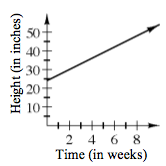### Home > CCA2 > Chapter Ch1 > Lesson 1.1.3 > Problem1-39

1-39.

Gregory planted a lemon tree in his back yard. When he planted the tree, it was $2$ feet tall. He noticed that it has been growing $3$ inches every week.

1. Create multiple representations ( $x → y$ table, graph, and equation) to represent the relationship between the days that have passed and the height of the tree.

Remember, there are $12$ inches in a foot. Start with a table.

How tall is the tree on day 0, the day it was planted?

Use what you know about how it grows to generate the rest of the table.

$\left. \begin{array} { c | c } { x } & { y } \\ \hline 0 & { 24 } \\ { 1 } & { 27 } \\ { 2 } & { 30 } \\ { 3 } & { 33 } \\ { 4 } & { 36 } \\ { 5 } & { 39 } \end{array} \right.$$y = 3x + 24$

2. If the tree continues growing at this rate, when will it be $6$ feet tall? How can you see this in each of the representations?

How many inches are there in $6$ feet?

Where do you see that on the table? On the graph? Describe it.

Substitute $72$ for $y$ in the equation and solve for $x$.

$16$ weeks.

3. State the possible inputs and outputs of the graph.

Possible inputs: all number greater than and including $0$. Possible outputs: all numbers greater than and including $24$.

Use the blank graph in the eTool below to help solve the problem.
Click the link at right for the full version of the eTool: CCA2 1-39 HW eTool